### Problem Set 7 Mensuration Class 10th Mathematics Part 2 MHB Solution

Problem Set 7

1. The ratio of circumference and area of a circle is 2:7. Find its circumference. Choose…
2. If measure of an arc of a circle is 160° and its length is 44 cm, find the…
3. Find the perimeter of a sector of a circle if its measure is 90° and radius is 7 cm.…
4. Find the curved surface area of a cone of radius 7 cm and height 24 cm. Choose the…
5. The curved surface area of a cylinder is 440 cm^2 and its radius is 5 cm. Find its…
6. A cone was melted and cast into a cylinder of the same radius as that of the base of…
7. Find the volume of a cube of side 0.01 cm. Choose the correct alternative answer for…
8. Find the side of a cube of volume 1 m^3 . Choose the correct alternative answer for…
9. A washing tub in the shape of a frustum of a cone has height 21 cm. The radii of the…
10. Some plastic balls of radius 1 cm were melted and cast into a tube. The thickness,…
11. A metal parallelopiped of measures 16 cm × 11cm × 10 cm was melted to make coins. How…
12. The diameter and length of a roller is 120 cm and 84 cm respectively. To level the…
13. The diameter and thickness of a hollow metals sphere are 12 cm and 0.01 m respectively.…
14. A cylindrical bucket of diameter 28 cm and height 20 cm was full of sand. When the sand…
15. The radius of a metallic sphere is 9 cm. It was melted to make a wire of diameter 4 mm.…
16. The area of a sector of a circle of 6 cm radius is 15π sq.cm. Find the measure of the…
17. In the figure 7.47, seg AB is a chord of a circle with centre P. If PA = 8 cm and…
18. In the figure 7.48, square ABCD is inscribed in the sector A- PCQ. The radius of…
19. In the figure 7.49, two circles with centre O and P are touching internally at point…

###### Problem Set 7
Question 1.

Choose the correct alternative answer for each of the following question.

The ratio of circumference and area of a circle is 2:7. Find its circumference.
A. 14π

B. 7/π

C. 7π

D.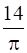Circumference of circle, C = 2πr

Area Of circle, A = πr2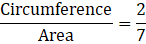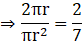⇒ r = 7

Circumference = 2× r× π

Circumference = 14π

∴ Option (A) is correct

Question 2.

Choose the correct alternative answer for each of the following question.

If measure of an arc of a circle is 160° and its length is 44 cm, find the circumference of the circle.
A. 66 cm

B. 44 cm

C. 160 cm

D. 99 cm

Measure of arc, θ = 160°

Length of arc, l = 44cm

As we know that,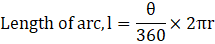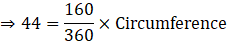⇒ Circumference = 99 cm

∴ Circumference of circle is 99cm, Option (D) is correct answer.

Question 3.

Choose the correct alternative answer for each of the following question.

Find the perimeter of a sector of a circle if its measure is 90° and radius is 7 cm.
A. 44 cm

B. 25 cm

C. 36 cm

D. 56 cm

Central angle, θ = 90°

As we know that,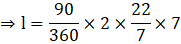⇒ l = 11cm

Perimeter of sector =Length of sector + (2× Radius)

Perimeter = 11 + (2 × 7)

Perimeter = 25cm

∴ Option (B) is correct.

Question 4.

Choose the correct alternative answer for each of the following question.

Find the curved surface area of a cone of radius 7 cm and height 24 cm.
A. 440 cm2

B. 550 cm2

C. 330 cm2

D. 110 cm2

Vertical Height, h = 24 cm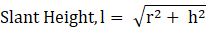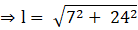⇒ l = 25 cm

Curved Surface Area of cone, A = πrl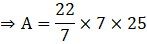⇒ A = 550 sq.cm

∴ Option (B) is correct.

Question 5.

Choose the correct alternative answer for each of the following question.

The curved surface area of a cylinder is 440 cm2 and its radius is 5 cm. Find its height.
A. 44/π cm

B. 22π cm

C. 44π cm

D. 22/π cm

Radius of cylinder, r = 5cm

Height of cylinder = h

Curved Surface Area of cylinder, A = 440 cm2 = 2πrh

440 = 2× π × 5 × h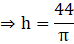∴ Option (A) is correct.

Question 6.

Choose the correct alternative answer for each of the following question.

A cone was melted and cast into a cylinder of the same radius as that of the base of the cone. If the height of the cylinder is 5 cm, find the height of the cone.
A. 15 cm

B. 10 cm

C. 18 cm

D. 5 cm

Height of cylinder, H = 5 cm

Height of cone =h

Volume of cylinder, V = πr2H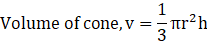Since cone is melted to form cylinder,

⇒ V = v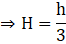⇒ h = 3× H

⇒ h = 3 × 5

⇒ h = 15 cm

∴ Option (A) is correct

Question 7.

Choose the correct alternative answer for each of the following question.

Find the volume of a cube of side 0.01 cm.
A. 1 cm3

B. 0.001 cm3

C. 0.0001 cm3

D. 0.000001 cm3

Side of the cube, a = 0.01 cm

Volume of cube, V = a3

⇒ V = (0.01)3

⇒ V = 0.000001 cm3

∴ Option (D) is correct

Question 8.

Choose the correct alternative answer for each of the following question.

Find the side of a cube of volume 1 m3.
A. 1 cm

B. 10 cm

C. 100 cm

D. 1000 cm

Let the side of cube be ‘a’ cm

Volume of cube, V = a3

⇒ As 1m3 = 106 cm3

⇒ a3 = 106

⇒ a = 100 cm

∴ Option (B) is correct

Question 9.

A washing tub in the shape of a frustum of a cone has height 21 cm. The radii of the circular top and bottom are 20 cm and 15 cm respectively. What is the capacity of the tub?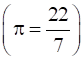Height of frustum, h = 21 cm

Two radii of frustum are, r1 = 20 cm and r2 = 15 cm

As we know,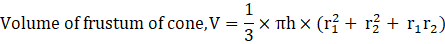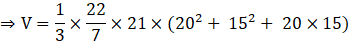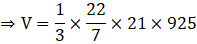⇒ V = 20350 cm3

⇒ V = 20.35 litres

∴ Capacity of bucket is 20.35 litres

Question 10.

Some plastic balls of radius 1 cm were melted and cast into a tube. The thickness, length and outer radius of the tube were 2 cm, 90 cm and 30 cm respectively. How many balls were melted to make the tube?

Volume of 1 plastic ball = VB

Radius of ball, rB = 1 cm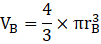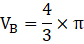Length of tube, h = 90 cm

Outer radius of tube, rO = 30 cm

Thickness of tube = 2 cm

Inner radius, rI = 30 – 2 = 28 cm

Volume of tube, VT = Volume of Outer cylinder – Volume of inner cylinder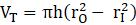⇒ VT = 90π(302 - 282)

⇒ VT = 90× 116 × π

Let ‘n’ be the number of balls melted.

⇒ VT = n × VB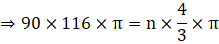⇒ n = 7830 balls

∴ 7830 balls were melted to make the tube.

Question 11.

A metal parallelopiped of measures 16 cm × 11cm × 10 cm was melted to make coins. How many coins were made if the thickness and diameter of each coin was 2 mm and 2 cm respectively?Volume of parallelepiped, VP = Product of its three dimensions

⇒ VP = 16 × 11 × 10

⇒ VP = 1760 cm3

Thickness of coin, t = 2mm = 0.2 cm

Diameter of coin = 2 cm

⇒ Radius of Coin, r = 1 cm

Volume of one coin, VC = πr2t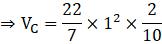⇒ VC = 0.6285 cubic cm

Let the number of coins made be ‘n’

⇒ VP = n × VC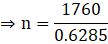⇒ n = 2800

Question 12.

The diameter and length of a roller is 120 cm and 84 cm respectively. To level the ground, 200 rotations of the roller are required. Find the expenditure to level the ground at the rate of Rs. 10 per sq. m

Diameter of roller = 120 cm

⇒ Radius, r = 60 cm

Length Of Roller, h = 84 cm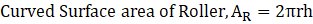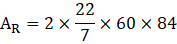⇒ AR = 31680 sq. cm

Total area of ground, AG = 200 × AR

⇒ AG = 6336000 cm2

As 1 m2 = 104 cm2

⇒ AG = 633.6 sq.m

Rate to level the ground = Rs. 10 per sq.m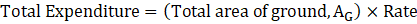⇒ Total Expenditure = 633.6 × 10

⇒ Total expenditure = Rs. 6336

∴ Total expenditure to level the road will be Rs. 6336

Question 13.

The diameter and thickness of a hollow metals sphere are 12 cm and 0.01 m respectively. The density of the metal is 8.88 gm per cm3. Find the outer surface area and mass of the sphere.

Thickness of sphere, t = 0.01m = 1 cm

Diameter of sphere = 12 cm

⇒ Outer Radius of sphere, RO = 6 cm

⇒ Inner Radius of sphere, RI = RO – t = 6 – 1 = 5 cm

Outer Surface area of sphere, A = 4πRO2

⇒ A = 4× 3.14 × 62

⇒ A = 452.16 sq.cm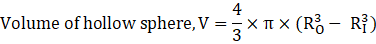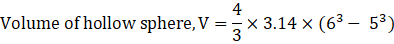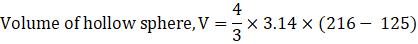⇒ V = 381.33 cm3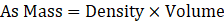⇒ Mass = 8.88 × 381.33

⇒ Mass = 3385.94 gm

∴ Outer surface area and mass of the sphere is 452.16 sq.cm and 3385.94 gm

Question 14.

A cylindrical bucket of diameter 28 cm and height 20 cm was full of sand. When the sand in the bucket was poured on the ground, the sand got converted into a shape of a cone. If the height of the cone was 14 cm, what was the base area of the cone?

Diameter of cylinder = 28 cm

⇒ Radius of cylinder, R = 14 cm

Height of cylinder, H = 20 cm

Volume of cylinder, VC = πR2H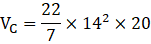Height of cone, h = 14 cm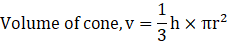Now, as the sand in cylinder forms the cone,

⇒ V = v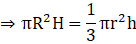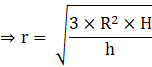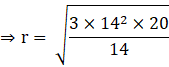⇒ r = 28.98 cm

Base area = πr2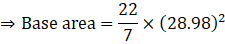⇒ Base area = 2640 sq. cm

∴ The base area of the cone formed is 2640 sq. cm

Question 15.

The radius of a metallic sphere is 9 cm. It was melted to make a wire of diameter 4 mm. Find the length of the wire.

Radius of sphere, R = 9 cm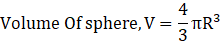Let the length of wire be H

Radius Of wire, r = 2 mm = 0.2 cm

Volume of wire , v = πr2H

As sphere is melted to make a wire,

⇒ V = v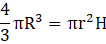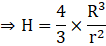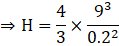⇒ H = 24300 cm

⇒ H = 243 m

∴ The length of the wire formed is 243 m

Question 16.

The area of a sector of a circle of 6 cm radius is 15π sq.cm. Find the measure of the arc and length of the arc corresponding to the sector.

Let the measure of arc be θ

Area of sector = 15π

As we know,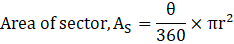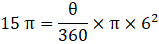⇒ θ = 150°

Also,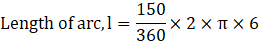⇒ l = 5π

∴ The length of the arc is equal to 5π.

Question 17.

In the figure 7.47, seg AB is a chord of a circle with centre P. If PA = 8 cm and distance of chord AB from the centre P is 4 cm, find the area of the shaded portion.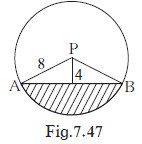Let ∠ APB = θ

Therefore,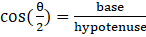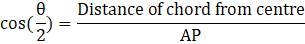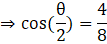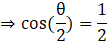⇒ θ = 120°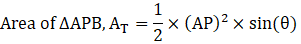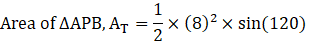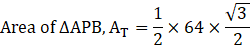⇒ AT = 27.68 sq.cm

Also,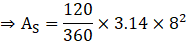⇒ AS = 66.96 sq.cm

Area of shaded region, AR = AS - AT

⇒ AR = 66.96 – 27.68

⇒ AR = 39.28 sq. cm

∴ area of shaded region is 39.28 sq. cm

Question 18.

In the figure 7.48, square ABCD is inscribed in the sector A- PCQ. The radius of sector C - BXD is 20 cm. Complete the following activity to find the area of shaded region.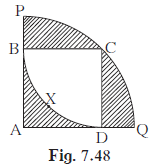Side of square ABCD = radius of sector C - BXD = 20 cm

Area of square = (side)2 = 2002 = 400 sq.cm

Area of shaded region inside the square = Area of square ABCD - Area of sector C - BXD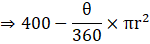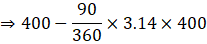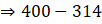⇒ 76 sq.cm

Radius of bigger sector = Length of diagonal of square ABCD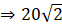Area of the shaded regions outside the square = Area of sector A - PCQ - Area of square ABCD = A(A - PCQ) - A(ABCD)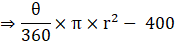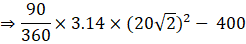⇒ 628 – 400

⇒ 228 sq.cm

∴ total area of the shaded region = 86 + 228 = 314 sq.cm.

Question 19.

In the figure 7.49, two circles with centre O and P are touching internally at point A. If BQ = 9, DE = 5, complete the following activity to find the radii of the circles.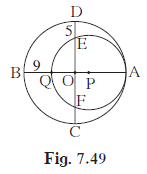Let the radius of the bigger circle be R and that of smaller circle be r.

OA, OB, OC and OD are the radii of the bigger circle

∴ OA = OB = OC = OD = R

PQ = PA = r

OQ = OB -BQ =R – 9

OE = OD - DE = R – 5

As the chords QA and EF of the circle with centre P intersect in the interior of the circle, so by the property of internal division of two chords of a circle,

OQ × OA = OE × OF

(R- 9) × R = (R- 5) × (R - 5) ……… (∵ OE = OF)

R2 - 9R = R2 - 10R + 25

R = 25

AQ = 2r = AB – BQ

2r = 50 - 9 = 41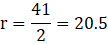## PDF FILE TO YOUR EMAIL IMMEDIATELY PURCHASE NOTES & PAPER SOLUTION. @ Rs. 50/- each (GST extra)

SUBJECTS

HINDI ENTIRE PAPER SOLUTION

MARATHI PAPER SOLUTION
SSC MATHS I PAPER SOLUTION
SSC MATHS II PAPER SOLUTION
SSC SCIENCE I PAPER SOLUTION
SSC SCIENCE II PAPER SOLUTION
SSC ENGLISH PAPER SOLUTION
SSC & HSC ENGLISH WRITING SKILL
HSC ACCOUNTS NOTES
HSC OCM NOTES
HSC ECONOMICS NOTES
HSC SECRETARIAL PRACTICE NOTES

2019 Board Paper Solution

HSC ENGLISH SET A 2019 21st February, 2019

HSC ENGLISH SET B 2019 21st February, 2019

HSC ENGLISH SET C 2019 21st February, 2019

HSC ENGLISH SET D 2019 21st February, 2019

SECRETARIAL PRACTICE (S.P) 2019 25th February, 2019

HSC XII PHYSICS 2019 25th February, 2019

CHEMISTRY XII HSC SOLUTION 27th, February, 2019

OCM PAPER SOLUTION 2019 27th, February, 2019

HSC MATHS PAPER SOLUTION COMMERCE, 2nd March, 2019

HSC MATHS PAPER SOLUTION SCIENCE 2nd, March, 2019

SSC ENGLISH STD 10 5TH MARCH, 2019.

HSC XII ACCOUNTS 2019 6th March, 2019

HSC XII BIOLOGY 2019 6TH March, 2019

HSC XII ECONOMICS 9Th March 2019

SSC Maths I March 2019 Solution 10th Standard11th, March, 2019

SSC MATHS II MARCH 2019 SOLUTION 10TH STD.13th March, 2019

SSC SCIENCE I MARCH 2019 SOLUTION 10TH STD. 15th March, 2019.

SSC SCIENCE II MARCH 2019 SOLUTION 10TH STD. 18th March, 2019.

SSC SOCIAL SCIENCE I MARCH 2019 SOLUTION20th March, 2019

SSC SOCIAL SCIENCE II MARCH 2019 SOLUTION, 22nd March, 2019

XII CBSE - BOARD - MARCH - 2019 ENGLISH - QP + SOLUTIONS, 2nd March, 2019

HSC Maharashtra Board Papers 2020

(Std 12th English Medium)

HSC ECONOMICS MARCH 2020

HSC OCM MARCH 2020

HSC ACCOUNTS MARCH 2020

HSC S.P. MARCH 2020

HSC ENGLISH MARCH 2020

HSC HINDI MARCH 2020

HSC MARATHI MARCH 2020

HSC MATHS MARCH 2020

SSC Maharashtra Board Papers 2020

(Std 10th English Medium)

English MARCH 2020

HindI MARCH 2020

Hindi (Composite) MARCH 2020

Marathi MARCH 2020

Mathematics (Paper 1) MARCH 2020

Mathematics (Paper 2) MARCH 2020

Sanskrit MARCH 2020

Important-formula

THANKS